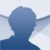Maxima

5.44.0

The so-called CAS (Computer Algebra Systems) allows you to solve symbolic algebra problems, using the power of the applications and computers. Thus, CAS applications ease the manipulations of symbolic and numeric expressions and their representation.

One of the best-known Computer Algebra Systems in university environments is Maxima, based on the legendary CAS developed by the MIT at the end of the '60s, Macsyma. One of its main features is that it's free and open-source, therefore, a long list of users is constantly working on its optimization

Main features

• Manipulation of all kinds of symbolic expressions: factorials, trigonometric functions, limits of expressions, differential calculus, integral calculus, matrix operations, differential equations, Laplace transforms, Taylor series, etc.
• Evaluation of numerical expressions with great precision.
• Graphic representation in 2D and 3D, and saving them in postscript format.

If you need a computational algebra system, download Maxima, an essential tool for mathematicians, physicists and university students.

Maxima
Over a year ago
More than a year ago
255 MB

We'd love to hear from you. Do you want to give us your opinion?Logged off